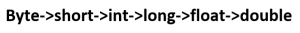# C# | Type Casting

### Prerequisite: C# Data Types

Type conversion happens when we assign the value of one data type to another. If the data types are compatible, then C# does Automatic Type Conversion. If not comparable, then they need to be converted explicitly which is known as Explicit Type conversion. For example, assigning an int value to a long variable.

### Implicit Type Casting / Automatic Type Conversion

It happens when:

• The two data types are compatible.
• When we assign value of a smaller data type to a bigger data type.

For Example, in C#, the numeric data types are compatible with each other but no automatic conversion is supported from numeric type to char or boolean. Also, char and boolean are not compatible with each other. Before converting, the compiler first checks the compatibility according to the following figure and then it decides whether it is alright or there some error.Following table shows the implicit types of conversion that is supported by C# :

Convert from Data Type Convert to Data Type
byte short, int, long, float, double
short int, long, float, double
int long, float, double
long float, double
float double

Example :

 `// C# program to demonstrate the ` `// Implicit Type Conversion ` `using` `System; ` `namespace` `Casting{ ` ` `  `class` `GFG { ` ` `  `        ``// Main Method ` `        ``public` `static` `void` `Main(String []args) ` `        ``{ ` `            ``int` `i = 57;  ` `             `  `            ``// automatic type conversion ` `            ``long` `l = i;  ` `              `  `            ``// automatic type conversion ` `            ``float` `f = l; ` `             `  `            ``// Display Result ` `            ``Console.WriteLine(``"Int value "`  `+i); ` `            ``Console.WriteLine(``"Long value "`  `+l); ` `            ``Console.WriteLine(``"Float value "`  `+f); ` `        ``} ` `} ` `} `

Output:

```Int value 57
Long value 57
Float value 57
```

### Explicit Type Casting

There may be compilation error when types not compatible with each other. For example, assigning double value to int data type:

 `// C# program to illustrate incompatible data  ` `// type for explicit type conversion ` `using` `System; ` `namespace` `Casting{ ` ` `  `class` `GFG { ` ` `  `        ``// Main Method ` `        ``public` `static` `void` `Main(String []args) ` `        ``{ ` `            ``double` `d = 765.12; ` ` `  `            ``// Incompatible Data Type ` `            ``int` `i = d; ` `             `  `            ``// Display Result     ` `            ``Console.WriteLine(``"Value of i is "``, +i); ` `        ``} ` `} ` `} `

Error :

```prog.cs(14,21): error CS0266: Cannot implicitly convert type `double' to `int'.
An explicit conversion exists (are you missing a cast?)
```

So, if we want to assign a value of larger data type to a smaller data type we perform explicit type casting.

• This is useful for incompatible data types where automatic conversion cannot be done.
• Here, target-type specifies the desired type to convert the specified value to.
• Sometimes, it may result into the lossy conversion.

Example :

 `// C# program to demonstrate the ` `// Explicit Type Conversion ` `using` `System; ` `namespace` `Casting{ ` ` `  `class` `GFG { ` ` `  `        ``// Main Method ` `        ``public` `static` `void` `Main(String []args) ` `        ``{ ` `            ``double` `d = 765.12; ` ` `  `            ``// Explicit Type Casting ` `            ``int` `i = (``int``)d; ` ` `  `            ``// Display Result ` `            ``Console.WriteLine(``"Value of i is "` `+i); ` `        ``} ` `} ` `} `

Output:

```Value of i is 765
```

Explanation :
Here due to lossy conversion, the value of i becomes 765 and there is a loss of 0.12 value.

#### C# provides built-in methods for Type-Conversions as follows :

Method Description
ToBoolean It will converts a type to Boolean value
ToChar It will converts a type to a character value
ToByte It will converts a value to Byte Value
ToDecimal It will converts a value to Decimal point value
ToDouble It will converts a type to double data type
ToInt16 It will converts a type to 16-bit integer
ToInt32 It will converts a type to 32 bit integer
ToInt64 It will converts a type to 64 bit integer
ToString It will converts a given type to string
ToUInt16 It will converts a type to unsigned 16 bit integer
ToUInt32 It will converts a type to unsigned 32 bit integer
ToUInt64 It will converts a type to unsigned 64 bit integer

Example :

 `// C# program to demonstrate the ` `// Built- In Type Conversion Methods ` `using` `System; ` `namespace` `Casting{ ` ` `  `class` `GFG { ` ` `  `        ``// Main Method ` `        ``public` `static` `void` `Main(String []args) ` `        ``{ ` `            ``int` `i = 12; ` `            ``double` `d = 765.12; ` `            ``float` `f = 56.123F; ` `             `  `            ``// Using Built- In Type Conversion ` `            ``// Methods & Displaying Result ` `            ``Console.WriteLine(Convert.ToString(f)); ` `            ``Console.WriteLine(Convert.ToInt32(d)); ` `            ``Console.WriteLine(Convert.ToUInt32(f)); ` `            ``Console.WriteLine(Convert.ToDouble(i)); ` `            ``Console.WriteLine(``"GeeksforGeeks"``); ` `        ``} ` `} ` `} `

Output:

```56.123
765
56
12
GeeksforGeeks
```

My Personal Notes arrow_drop_upCheck out this Author's contributed articles.

If you like GeeksforGeeks and would like to contribute, you can also write an article using contribute.geeksforgeeks.org or mail your article to contribute@geeksforgeeks.org. See your article appearing on the GeeksforGeeks main page and help other Geeks.

Please Improve this article if you find anything incorrect by clicking on the "Improve Article" button below.

Article Tags :

2

Please write to us at contribute@geeksforgeeks.org to report any issue with the above content.